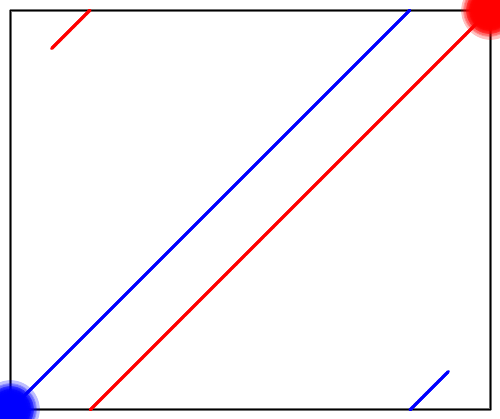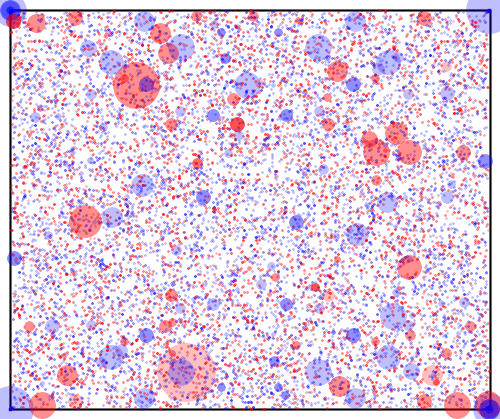Small Squares

There are many algorithms for finding the prime factors of a large integer n, but the most effective are based on the simple idea of finding integers x and y satisfying x2y2 (mod n). Then (x + y)(xy) ≡ 0 (mod n) and there is a good chance that gcd(x + y, n) will be a non-trivial factor of n. Different factorization algorithms use different methods to find x and y that have the same square modulo n, ranging from Fermat’s method which can be worse than trial division to the number field sieve which is the best known factoring algorithm. A simple method is to randomly choose an integer x in the range √n < x < n and check whether x2 mod n is a square integer y2.

Example: This article will use as a running example the 32-bit integer n = 2,768,439,589 which is the product of the two primes p = 57,641 and q = 48,029. Suppose x is randomly chosen to be 267,857,730. Then x2 mod n = 9, and therefore x2 ≡ 32 (mod n) and a non-trivial factor is recovered by gcd(x + 3, n) = q. □

The simple method can be greatly improved by using a sieve, which relaxes the requirement that x2 mod n is a square, and instead requires it to be a product of elements of a factor base FB = {-1} ∪ { p | p is a prime and pB }. Integers satisfying this condition are called B-smooth, and after discovering |FB| + 1 B-smooth integers of the form xi2 mod n linear algebra can be used to find a subset I ⊆ {1, …, |FB| + 1} such that ΠiI (xi2 mod n) is a square integer y2. Then (ΠiI xi)2y2 (mod n) as desired.

Example: Let F11 = {-1,2,3,5,7,11} and suppose the following 11-smooth integers have been discovered:

• If x1 = 32,051,026 then x12 mod n = -20 = (-1)122305170110
• If x3 = 135,988,713 then x32 mod n = -210 = (-1)121315171110
• If x7 = 373,858,046 then x72 mod n = 42 = (-1)021315071110

Now modulo n

• (x1x3x7)2
• ≡  (x12 mod n)(x32 mod n)(x72 mod n)
• ≡  ((-1)122305170110)((-1)121315171110)((-1)021315071110)
• ≡  ((-1)224325272110)
• ≡  ((-1)122315171110)2
• ≡  (-420)2

and a non-trivial factor is again recovered by gcd(x1x3x7 – 420, n) = q. □

Since numbers with small absolute value are more likely to be B-smooth, most factorization algorithms using this approach are based on a particular method for generating integers x such that x2 mod n has small absolute value. The remainder of this article will describe a few different methods and a way to visualize the generated integers.

Visualizing Numbers

By the Chinese Remainder Theorem there is a one-to-one correspondence between numbers x mod n ∈ {0, …, n – 1} and pairs of numbers (x mod p, x mod q) ∈ {0, …, p – 1} × {0, …, q – 1}. Using this correspondence a number x mod n can be plotted as a circle inside a box of size p×q. The circle is colored blue for positive numbers (i.e., 2(x mod n) < n), and red for negative numbers (i.e., 2(x mod n) ≥ n). The size of the circle increases as the absolute value of x mod n decreases (where the absolute value of a number x mod n is itself if it is positive, and -x mod n if it is negative).

Example: Here is a plot of all numbers x mod n where the integer x satisfies -√n < x < √n. □Since the goal is to find integers x such that x2 mod n has small absolute value, the visualization is changed to communicate both pieces of information. The position of the circle plotted for the number x mod n is calculated as above, but the color and size of the circle is calculated from the sign and absolute value of x2 mod n.

Example: Here is a plot of all 1,352 numbers x mod n such that the absolute value of x2 mod n is at most 634. □The reflective symmetric pattern occurs because every square number x2 mod n is the square of four numbers with circles plotted at the following positions:

• (x mod p, x mod q)
• (x mod p, –x mod q)
• (-x mod p, x mod q)
• (-x mod p, –x mod q)

This provides a visual interpretation of the core factorization approach: given two of these circles that are not diagonally opposite each other, adding them will produce a point that is on the edge of the box, and the edges consist of all the integers that have a nontrivial common divisor with n.

The blue corners are the result of the integers {1, 2, …} having small positive squares. This makes the bottom left corner blue, and the reflective symmetry means that what happens in one corner happens in them all.

Beyond these features, it is striking how random the visualization appears: finding numbers with small squares is a number theory version of finding a needle in a haystack. The following three sections will each describe an algorithm for searching these kind of haystacks.

Square Roots

The first algorithm for finding integers x such that x2 mod n has small absolute value proceeds by checking integers around √(kn) for small k ∈ {1, 2, …}, since for i ∈ {0, 1, …}

• |(⌈√(kn)⌉ + i)2 mod n|
• =  |(√(kn) + (i + (⌈√(kn)⌉ – √(kn))))2 mod n|
• =  |(kn + 2(i + (⌈√(kn)⌉ – √(kn)))√(kn) + (i + (⌈√(kn)⌉ – √(kn)))2) mod n|
• =  |(2(i + (⌈√(kn)⌉ – √(kn)))√(kn) + (i + (⌈√(kn)⌉ – √(kn)))2) mod n|
• ≤  2(i + (⌈√(kn)⌉ – √(kn)))√(kn) + (i + (⌈√(kn)⌉ – √(kn)))2
• ≤  2(i + 1)√(kn) + (i + 1)2

and similarly

• |(⌊√(kn)⌋ – i)2 mod n|  ≤  2(i + 1)√(kn) + (i + 1)2

Minimizing the absolute value of x2 mod n is thus achieved by choosing integers x from a range of the form √(kn) – m < x < √(kn) + m.

Example: Here is a plot of all 20,000 integers x in the ranges √(kn) – m < x < √(kn) + m, where k ∈ {1, …, 20} and m = 500.The plot of each range around √(kn) looks like a battleship that is painted blue for the numbers greater than √(kn) and red for the numbers less than √(kn). Unfortunately, none of the numbers on the battleships are marked as larger “hits”, meaning that the absolute value of all of the squares is greater than 634. □

Checking integers close to √(kn) may seem like a simple idea, but the quadratic sieve does exactly this and is the second fastest factorization algorithm after the number field sieve. The quadratic sieve works so well because it defines another sieve algorithm that can search whole ranges at a time to identify all integers x such that x2 mod n are smooth numbers.

Continued Fractions

The second algorithm for finding integers x such that x2 mod n has small absolute value is based on continued fractions. For any real number r, its continued fraction approximation is a sequence of rationals ai/bi each having the property that

• |ai/bir|  ≤  1/bi2

If a/b is a continued fraction approximation to √n, then

• |a/b – √n|  ≤  1/b2
• ⇔  √n – 1/b2  <  a/b  <  √n + 1/b2
• ⇔  bn – 1/b  <  a  <  bn + 1/b
• ⇔  b2n – 2√n + 1/b2  <  a2  <  b2n + 2√n + 1/b2
• ⇔  -2√n + 1/b2  <  a2b2n  <  2√n + 1/b2
• ⇒  |a2b2n|  <  2√n + 1/b2
• ⇒  |a2 mod n|  <  2√n + 1/b2

The continued fraction factorization algorithm computes the sequence of continued fraction approximations ai/bi to √n and searches for smooth numbers in ai2 mod n.

Example: Here is a plot of the first 20,000 numerators ai in the sequence of continued fraction approximations to √n.The visualization clearly shows two differences between the continued fraction factorization algorithm and the quadratic sieve. Firstly, the generated numbers ai mod n are not clustered in groups, but rather are scattered throughout the whole range {0, …, n – 1}. Secondly, the effect of the tighter bound on the absolute value of ai2 mod n can be observed by the presence of larger circles (each marking an absolute value of at most 634). □

The Pell Equation

The third algorithm for finding integers x such that x2 mod n has small absolute value is based on the Pell equation. Given a positive integer n the Pell equation is a2 = nb2 + 1, and the goal is to find all integers a and b that solve the equation. If n is a square, then every solution must correspond to two integer squares that are distance 1 apart, which can only occur in the trivial solution a = ±1 and b = 0. If n is not a square, then there are infinitely many integer solutions which can all be derived from the smallest nontrivial solution computed by the Chakravala method. The Chakravala method generates a sequence of triples (ai, bi, ki) that satisfy the equation ai2 = nbi2 + ki. Each triple is used to generate the next triple according to a fixed rule which sets up a condition and then finds the minimal |ki| that satisfies the condition. The initial triple is (a0, b0, k0) = (m, 1, m2n) where m is chosen to be whichever of ⌊√n⌋ and ⌈√n⌉ minimizes |k0|. The sequence is guaranteed to converge to the smallest nontrivial integer solution of the Pell equation, which is the values of ai and bi when ki = 1.

Example: Given the Pell equation a2 = 61b2 + 1, here is the sequence computed by the Chakravala method:

• [(8,1,3), (39,5,-4), (164,21,-5), (453,58,5), (1523,195,4), (5639,722,-3), (29718,3805,-1), (469849,60158,-3), (2319527,296985,4), (9747957,1248098,5), (26924344,3447309,-5), (90520989,11590025,-4), (335159612,42912791,3), (1766319049,226153980,1)]

The smallest nontrivial integer solution a = 1,766,319,049 and b = 226,153,980 can be read from the last triple in the sequence. □

Note that each triple (ai, bi, ki) generated by the Chakravala method satisfies ai2ki (mod n), so the Chakravala method can be turned into a factorization algorithm by generating triples (ai, bi, ki) and searching for smooth numbers in ai2 mod n.

Example: Here is a plot of the first 20,000 integers ai from triples (ai, bi, ki) generated by the Chakravala method.The visualization is similar to the one for the numerators of the continued fraction approximations, covering the whole range {0, …, n – 1} and containing larger circles marking that the absolute value of the square is at most 634. However, closer examination shows that the Pell equation visualization is overall darker than the continued fraction visualization, indicating that the Chakravala method is finding more small squares than the continued fraction approximations. Perhaps the idea of turning the Chakravala method into a factorization algorithm is worth further study. □

References

All of the computations and images in this article were generated using Haskell.